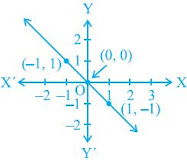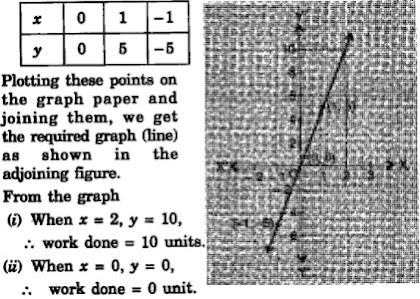# NCERT Solutions for Chapter 4 Linear Equations in Two Variables Class 9 Maths

 Chapter Name NCERT Solutions for Chapter 4 Linear Equations in Two Variables Class Class 9 Topics Covered Linear Equation in one VariableLinear Equation in two VariablesGraph of Linear Equations in two Variables Related Study Materials NCERT Solutions for Class 9 MathsNCERT Solutions for Class 9Revision Notes for Chapter 4 Linear Equations in Two Variables Class 9 MathsImportant Questions for Chapter 4 Linear Equations in Two Variables Class 9 MathsMCQ for for Chapter 4 Linear Equations in Two Variables Class 9 Maths

## Short Revision for Ch 4 Linear Equations in Two Variables Class 9 Maths

1. An equation of the form ax + by + c = 0, where a, b and c are real numbers, such that a and b are not both zeroes, is called a linear equation in two variables x and y.
2. A linear equation in two variables has infinitely many solutions.
3. A solution of ax + by + c = 0 is an ordered pair (x, y).
4. The graph of a linear equation is obtained by joining any two of its solutions.
5. The graph of a linear equation in two variables is a straight line.
6. All the ordered pairs lie on the straight line.
7. The equation of x-axis is y = 0 and that of y-axis is x = 0.
8. A line parallel to x-axis is perpendicular to y-axis and parallel to y-axis is perpendicular to x-axis.
9. The equation of any line passing through the origin is of type y = mx.
10. Every point on the graph of a linear equation in two variables is a solution of the linear equation.

### Exercise 4.1

1. The cost of a notebook is twice the cost of a pen. Write a linear equation in two variables to represent this statement.
(Take the cost of a notebook to be ₹ x and that of a pen to be ₹ y).

Solution

Let cost of a notebook = ₹ x and cost of a pen = ₹ y.
According to given condition,
x = 2y

⇒ x - 2y = 0

2. Express the following linear equations in the form ax + by + c = 0 and indicate the values of a, b and c in each case:
(i) 2x + 3y = 9.3
5
(ii) x –(y/5)–10 = 0
(iii) –2x+3y = 6
(iv) x = 3y
(v) 2x = –5y
(vi) 3x+2 = 0
(vii) y–2 = 0
(viii) 5 = 2x

### Exercise 4.2

1. Which one of the following options is true, and why?
y = 3x+5 has
(i) A unique solution
(ii) Only two solutions
(iii) Infinitely many solutions

Solution

(iii) As each linear equation in two variable has infinitely many solutions. Further, for every x there is a corresponding value of y and vice - versa.

2. Write four solutions for each of the following equations:
(i) 2x+y = 7
(ii) πx+y = 9
(iii) x = 4y

3. Check which of the following are solutions of the equation x–2y = 4 and which are not:
(i) (0, 2)
(ii) (2, 0)
(iii) (4, 0)
(iv) (√2, 4√2)
(v) (1, 1)

4. Find the value of k, if x = 2, y = 1 is a solution of the equation 2x+3y = k.

Solution

If x = 2, y = 1 is a solution of the equation 2x + 3y = k, then 4 + 3 = k ⇒ 7.

### Exercise 4.3

1. Draw the graph of each of the following linear equations in two variables:
(i) x + y = 4
(ii) x–y = 2
(iii) y = 3x
(iv) 3 = 2x + y

2. Give the equations of two lines passing through (2, 14). How many more such lines are there, and why?

3. If the point (3, 4) lies on the graph of the equation 3y = ax+7, find the value of a.

Solution

If point (3, 4) lies on the graph of the equation 3y = ax + 7, then the point satisfies this equation.
∴ 12 = 3a + 7 ⇒ 3a = 5 ⇒ a = 5/3.

4. The taxi fare in a city is as follows: For the first kilometer, the fare is 8 and for the subsequent distance it is 5 per km. Taking the distance covered as x km and total fare as  y, write a linear equation for this information, and draw its graph.

5. From the choices given below, choose the equation whose graphs are given in Fig. (1) and Fig. (2).
For Fig. (1)
(i) y = x
(ii) x + y = 0
(iii) y = 2x
(iv) 2 + 3y = 7xFor Fig. (2)
(i) y = x + 2
(ii) y = x - 2
(iii) y = -x + 2
(iv) x + 2y = 6

6. If the work done by a body on application of a constant force is directly proportional to the distance travelled by the body, express this in the form of an equation in two variables and draw the graph of the same by taking the constant force as 5 units. Also read from the graph the work done when the distance travelled by the body is
(i) 2 units
(ii) 0 unit

Solution

Let y units be the work done by a body on application of a constant force of 5 units and the distance travelled be x units.
Therefore, we have y = 5x as the required equation.
Some points on graph are :7. Yamini and Fatima, two students of Class IX of a school, together contributed ₹ 100 towards the Prime Minister’s Relief Fund to help the earthquake victims. Write a linear equation which satisfies this data. (You may take their contributions as ₹ x and ₹ y.) Draw the graph of the same.

8. In countries like USA and Canada, temperature is measured in Fahrenheit, whereas in countries like India, it is measured in Celsius. Here is a linear equation that converts Fahrenheit to Celsius :
F = (9/5)C + 32
(i) Draw the graph of the linear equation above using Celsius for x-axis and Fahrenheit for y-axis.
(ii) If the temperature is 30°C, what is the temperature in Fahrenheit?
(iii) If the temperature is 95°F, what is the temperature in Celsius?
(iv) If the temperature is 0°C, what is the temperature in Fahrenheit and if the temperature is 0°F, what is the temperature in Celsius?
(v) Is there a temperature which is numerically the same in both Fahrenheit and Celsius? If yes, find it.

1. Give the geometric representations of y = 3 as an equation
(i) in one variable
(ii) in two variables

2. Give the geometric representations of 2x+9 = 0 as an equation
(i) in one variable
(ii) in two variables